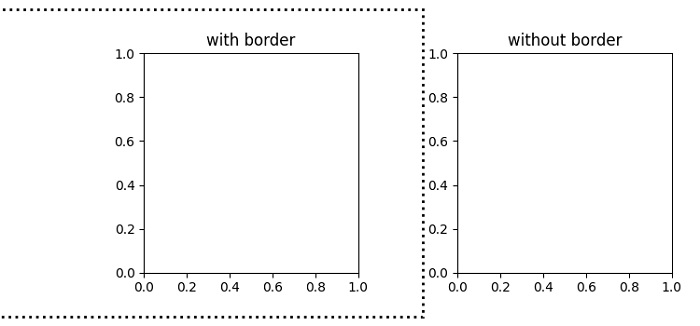# Draw a border around subplots in Matplotlib

To draw a border around subplots in matplotlib, we can use a Rectangle patch on the subplots.

## Steps

• Set the figure size and adjust the padding between and around the subplots.

• Add a subplot to the current figure using subplot(121).

• Get the subplot axes.

• Add a rectangle defined via an anchor point *xy* and its *width* and *height*.

• Add a rectangle patch to the current subplot based on axis (Step 4).

• Set whether the artist uses clipping.

• Add a subplot to the current figure using subplot(122).

• Set the title of the current subplot.

• To display the figure, use show() method.

## Example

from matplotlib import pyplot as plt

plt.rcParams["figure.figsize"] = [7.50, 3.50]
plt.rcParams["figure.autolayout"] = True

sub = plt.subplot(121)
ax = sub.axis()
rec = plt.Rectangle((ax - 0.7, ax - 0.2), (ax - ax) + 1, (ax - ax) + 0.4, fill=False, lw=2, linestyle="dotted")

rec.set_clip_on(False)
plt.title("with border")

sub = plt.subplot(122)
plt.title("without border")

plt.show()

## Output